# NCERT Solutions for Class 10th Maths Chapter 3 : Linear Equations

In this page we have NCERT Solutions for Class 10th Maths:Linear Equations for Exercise 3.1 on page 44. Hope you like them and do not forget to like , social_share and comment at the end of the page.
A pair of linear equations in two variables can be represented, and solved, by the:
(i) graphical method
(ii) algebraic method
How to draw the graph of the linear equation
1. Suppose the equation given is
$ax+by+c=0 \; , \; a \neq 0 \; and \; b \neq 0$
2. Find the value of y for x=0
$y=\frac {-c}{b}$
This point will lie on Y –axis. And the coordinates will be $(0,\frac{-c}{b})$
3. Find the value of x for y=0
$x=\frac {-c}{a}$
This point will lie on X –axis. And the coordinates will be $(\frac {-c}{a}, 0)$
4. Now we can draw the line joining these two points
5. If the points from above does not corresponds to integers, we can choose points where values are integers

## NCERT Solutions for Class 10th Maths Chapter 3 Exercise 3.1

Question 1.
Aftab tells his daughter, “Seven years ago, I was seven times as old as you were then. Also, three years from now, I shall be three times as old as you will be.” (Isn’t this  interesting?) Represent this situation algebraically and graphically.
Solution 1:
Let  age of Aftab is represented by x
And age of daughter is represented by y
Then Seven years ago,
Age of Aftab = $x -7$
Age of daughter= $y-7$
As per the question Aftab  was seven times as old as her daughter then were  so we get
$(x - 7) = 7 (y - 7)$
$x - 7y = -42$ ----(1)
Now Three years from now ,
Age of Aftab = $x +3$
Age of daughter = $y+3$
As per question Aftab  shall be three times as old as her daughter will be so we get
$x + 3 = 3 (y + 3)$
$x -3y = 6$ ----- (2)
Algebraic representation  of situation
$x - 7y = -42$ ----(1)
$x -3y = 6$ ----- (2)
To represent it graphically, we need to plot these equation  on the XY coordinate system.
We can choose two values of each equation and plot it on XY coordinate system
$x - 7 y = - 42$ or
$y=\frac {(x+42)}{7}$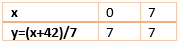Now
$x -3 y = 6$
$y=\frac {(x-6)}{3}$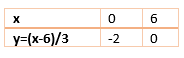We can plot these two lines on XY coordinate system using the two point derived above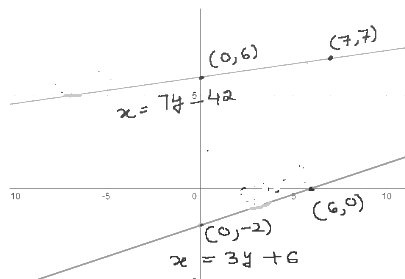Question 2.
The coach of a cricket team buys 3 bats and 6 balls for Rs 3900. Later, she buys another bat and 3 more balls of the same kind for Rs 1300. Represent this situation algebraically and geometrically.
Solution 2:
Let Cost of one bat    = Rs x
Let Cost of one ball    = Rs y
3 bats and 6 balls for Rs 3900 So that
$3 x + 6y = 3900$ ---- (1)
Given that she buys another bat and 2 more balls of the same kind for Rs 1300
So we get
$x + 2 y = 1300$ ---(2)
Algebraic representation  of situation
$3 x + 6y = 3900$ ---- (1)
$x + 2 y = 1300$ ---(2)
To represent it graphically, we need to plot these equation  on the XY coordinate system.
We can choose two values of each equation and plot it on XY coordinate system
$3 x + 6y = 3900$
or
$y=\frac {(3900-3x)}{6}$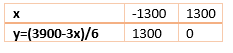$x +2 y = 1300$
$y=\frac {(1300-x)}{2}$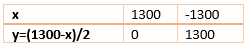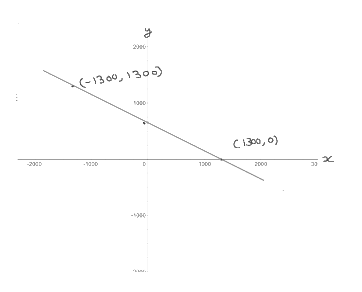Question 3.
The cost of 2 kg of apples and 1kg of grapes on a day was found to be Rs 160. After a month, the cost of 4 kg of apples and 2 kg of grapes  is Rs300.   . Represent this situation algebraically and geometrically.
Solution 3:
Let cost each kg of apples   = Rs x
Cost of each kg of grapes    = Rs y
The cost of 2 kg of apples and 1kg of grapes on a day was found to be Rs 160
So that
$2 x + y = 160$ ----- (1)
Given that the cost of 4 kg of apples and 2 kg of grapes is Rs 300
so we get
$4x + 2y= 300$ --- (2)
Algebraic representation  of situation
$2 x + y = 160$ ----- (1)
$4x + 2y= 300$ --- (2)
To represent it graphically, we need to plot these equation  on the XY coordinate system.
We can choose two values of each equation and plot it on XY coordinate system
$2x +y = 160$
or
$y=160-2x$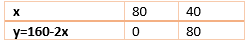$4x + 2y= 300$
$y=\frac {(300-4x)}{2}$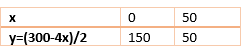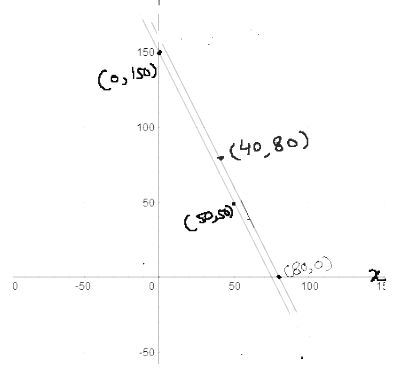## Summary

1. NCERT Solutions for Class 10 Maths Chapter 3 Linear Equations Exercise 3.1 has been prepared by Expert with utmost care. If you find any mistake.Please do provide feedback on mail. You can download the solutions as PDF in the below Link also
2. This chapter 3 has total 7 Exercise 3.1 ,3.2,3.3,3.4,3.5,3.6 and 3.7. This is the First exercise in the chapter.You can explore previous exercise of this chapter by clicking the link belowGo back to Class 10 Main Page using below links

### Practice Question

Question 1 What is $1 - \sqrt {3}$ ?
A) Non terminating repeating
B) Non terminating non repeating
C) Terminating
D) None of the above
Question 2 The volume of the largest right circular cone that can be cut out from a cube of edge 4.2 cm is?
A) 19.4 cm3
B) 12 cm3
C) 78.6 cm3
D) 58.2 cm3
Question 3 The sum of the first three terms of an AP is 33. If the product of the first and the third term exceeds the second term by 29, the AP is ?
A) 2 ,21,11
B) 1,10,19
C) -1 ,8,17
D) 2 ,11,20